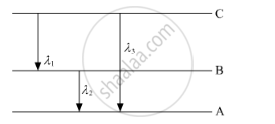# State Bohr'S Quantization Condition for Defining Stationary Orbits. - Physics

(i) State Bohr's quantization condition for defining stationary orbits. How does the de Broglie hypothesis explain the stationary orbits?

(ii) Find the relation between three wavelengths λ1, λ2 and λ3 from the energy-level diagram shown below.#### Solution

(a) Bohr's quantisation condition: According to Bohr, an electron can revolve only in certain discrete, non-radiating orbits for which total angular momentum of the revolving electron is an integral multiple of h/(2pi)where h is the Planck's constant.

:.mvr=(nh)/(2pi)

(b) Using the Rydberg formula of the spectra of hydrogen atom, we write

1/lamda_1=R(1/n_(2^2)-1/n_(3^2)) ......(1)

1/lamda_2=R(1/n_(1^2)-1/n_(2^2)) ......(2)

1/lamda_3=R(1/n_(1^2)-1/n_(3^2)) ......(3)

Adding (1) and (2), we find that

1/lambda_1+1/(lambda_2)=R(1/n_(1^2)-1/n_(3^2))=1/lambda_3

This is the required relation between the three wavelengths.

Concept: Bohr’s Model for Hydrogen Atom
Is there an error in this question or solution?
2015-2016 (March) Delhi Set 1

Share